# Electronics and Communication Engineering - Exam Questions Papers

31.

The common emitter model is shown below: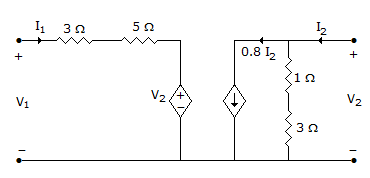The h-parameter of this model are:

 A.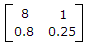B.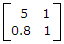C.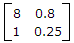D.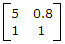Explanation:

h parameter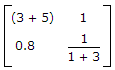V1 = (3 + 5)I1 + V2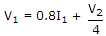.

32.

In the following limiter circuit, an input voltage V1 = 10 sin 100pt applied. Assume that the diode drop is 0.7 V when it is forward biased. The zener breakdown voltage is 6.8 V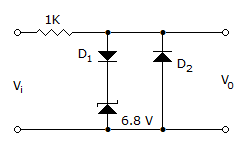The maximum and minimum values of the output voltage respectively are

 A. 6.1 V, -0.7 V B. 0.7 V, -7.5 V C. 7.5 V, -0.7 V D. 7.5 V, -7.5 V

Explanation:

During +ve part of Vi

D1 will be forward biased

Zener diode is reverse biased

Thus net voltage = 6.8 + 0.7 = 4.5 V

During -ve part of Vi

D2 will be forward biased

D1 will be reversed biased

Thus net voltage = -0.7 V .

33.

Four signals
m1 = cos (ω0t), m2(t) =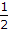cos (ω0t), m3(t) = 2 cos (ω0t) and m4(t) = cos (4 ω0t) are multiplexed by Time Division Multiplexing system. The commutator speed in rps is

 A. 2f0 B. 3f0 C. 4f0 D. 8f0

Explanation:

Apply Nyquist criteria.

Maximum angular speed is 4ω0

Thus commutator speed must be 2 x 4ω2 = 8ω0 .

34.

The feedback control system shown in figure is stable for what values of K and T?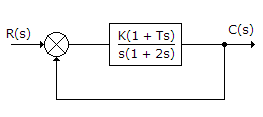A. K < 0, T > - 1 B. K > 0, T > 0 C. K > 0, T > - 1 D. K < 0, T > 0

Explanation:

Apply Routh Hurwitz criteria.

35.

Find z parameters for the following network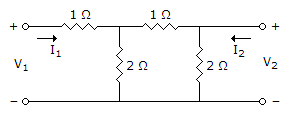A.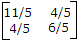B.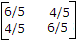C.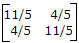D.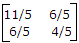Explanation:

Use Mesh analysis

z parameters are given by,

V1 = Z11I1 + Z12I2 ...(a)

V2 = Z21I1 + Z22I2 ...(b)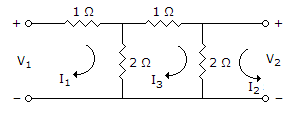Apply KVL to mesh 1

V1 = 3I1 - 2I3 ...(i)

Apply KVL to mesh 2

V2 = 2I2 + 2I3 ...(ii)

Apply KVL to mesh 3

5I3 + 2I2 -2I1 =0 ...(iii)

5I3 = 2I1 - 2I2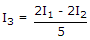Put I3 in eq. (i)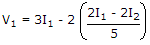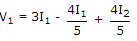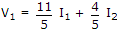...(iv)

Put in (ii)

Similarly,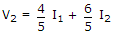...(v)

Compare equation (iv) to (a) and equation (v) to (b)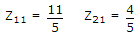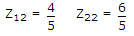.

#### Current Affairs 2022

Interview Questions and Answers